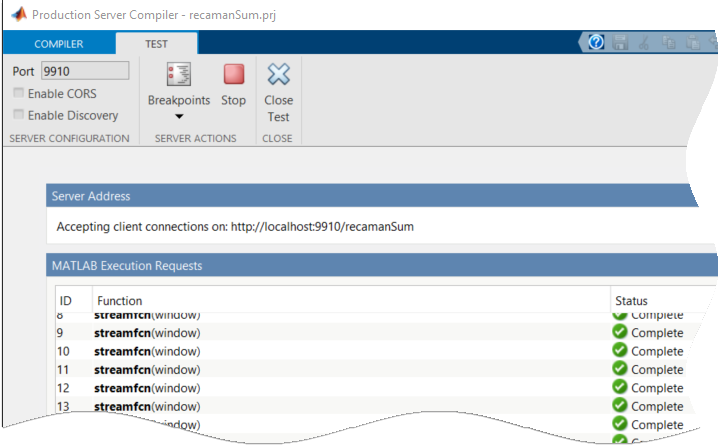## Test Streaming Analytic Function Using Local Test Server

This example shows how to use the development version of MATLAB® Production Server™ to test a streaming analytic function before deployment to MATLAB Production Server. MATLAB Compiler SDK™ includes the development version of MATLAB Production Server, which you can use as a local test server for testing and debugging application code before deploying it to enterprise systems.

### Prerequisites

• You must have Streaming Data Framework for MATLAB Production Server installed on your system. For more information, see Install Streaming Data Framework for MATLAB Production Server.

• You must have a running Kafka® server where you have the necessary permissions to create topics. The example assumes that the network address of your Kafka host is `kafka.host.com:9092`.

### Write Streaming Analytic MATLAB Function

For testing purposes, use the sample MATLAB functions `recamanSum` and `initRecamanSum` located in the `support_package_root\mps\streaming\Examples\Numeric` folder, where `support_package_root` is the root folder of support packages on your system. To get the path to this folder, use this command:

`fullfile(matlabshared.supportpkg.getSupportPackageRoot,'toolbox','mps','streaming','Examples','Numeric')`

Later, you test the `recamanSum` deployable archive using the local test server.

For details about the `recamanSum` and `initRecamanSum` functions and to access the code, see Write Stateful Function and Write State Initialization Function.

### Create Sample Streaming Data

Prepare for testing by creating sample data and writing the data to a Kafka stream. For this example, you create a 1000-element Recamán sequence and write it to a Kafka topic `recamanSum_data`.

To create the Recamán sequence, you can use the following `recamanTimeTable` function, which is also located in the `\Examples\Numeric` folder. `recamanTimeTable` creates a timetable containing the first `N` elements of a Recamán sequence.

```function tt = recamanTimeTable(N) rs = zeros(1,N); for k=2:N n = k-1; subtract = rs(k-1) - n; if subtract > 0 && any(rs == subtract) == false rs(k) = subtract; else rs(k) = rs(k-1) + n; end end incr = seconds(1:N); thisVeryInstant = ... convertTo(datetime, "epochtime", "Epoch", "1970-1-1"); thisVeryInstant = datetime(thisVeryInstant, "ConvertFrom",... "epochtime", "Epoch", "1970-1-1"); thisVeryInstant.TimeZone = "UTC"; timestamp = (thisVeryInstant - seconds(N)) + incr'; key = (0:N-1)'; key = string(key); R = rs'; tt = timetable(timestamp,R,key); end```

You can use the following code to create a 1000-element Recamán sequence using the `recamanTimeTable` function and write it to the `recamanSum_data` Kafka topic. The example assumes that you have Kafka host running at the network address `kafka.host.com:9092` and you have the necessary permissions to create topics in the Kafka cluster.

```kafkaHost = "kafka.host.com"; kafkaPort = 9092; tt0 = recamanTimeTable(1000); dataKS = kafkaStream(kafkaHost, kafkaPort, "recamanSum_data", Rows=100); try deleteTopic(dataKS); catch, end writetimetable(dataKS, tt0); tt1 = readtimetable(dataKS); if isequal(tt0(1:height(tt1),:), tt1) fprintf(1,"Success writing data to topic %s.\n", dataKS.Name); end stop(dataKS); ```

### Simulate Production Using Local Test Server

To simulate streaming data processing in a production environment, you can run the `recamanSum` deployable archive using the development version of MATLAB Production Server and process data from the `recamanSum_data` topic.

1. Create a `KafkaStream` object connected to the `recamanSum_data` topic.

`ks = kafkaStream("kafka.host.com",9092,"recamanSum_data");`
2. Create another `KafkaStream` object to write the results of the `recamanSum` analytic function to a different topic called `recaman_results`.

`outKS = kafkaStream("kafka.host.com",9092,"recamanSum_results");`
3. Create an `EventStreamProcessor` object that runs the `recamanSum` function and initializes persistent state with the `initRecamanSum` function.

Providing a persistent data storage connection name as an input argument is optional. If you do not provide one, `EventStreamProcessor` creates a connection with a unique name to cache the data state between iterations.

`esp = eventStreamProcessor(ks,@recamanSum,@initRecamanSum,OutputStream=outKS);`
4. Using the MATLAB editor, you can set breakpoints in the `recamanSum` function to examine the incoming streaming data when you start the server.

5. Start the test server.

`startServer(esp);`

Doing so opens the Production Server Compiler app with values for the streaming function `recamanSum`, the entry point function `streamfcn`, and the deployable archive `recamanSum`.6. Start the test server from the app by clicking Test Client, and then Start.

7. Navigate back to the MATLAB command prompt to start processing events.

`start(esp);`

In the Production Server Compiler app, you can see that the test server receives data.8. From the MATLAB editor, if you had set breakpoints, you can use the debugger to examine the data, state, and results of the function processing. Click Continue to continue debugging or Stop when you have finished debugging.

9. From the MATLAB command prompt, stop event processing and shut down the server.

```stop(esp); stopServer(esp);```
10. Read the processed results from the output stream.

`results = readtimetable(outKS);`
```results = 50×2 timetable timestamp key sum ____________________ ____ ____ 20-Jan-1970 04:55:08 "0" 0 20-Jan-1970 04:55:08 "1" 1 20-Jan-1970 04:55:08 "2" 4 20-Jan-1970 04:55:08 "3" 10 20-Jan-1970 04:55:08 "4" 12 : : : 20-Jan-1970 04:55:08 "45" 1697 20-Jan-1970 04:55:08 "46" 1732 20-Jan-1970 04:55:08 "47" 1814 20-Jan-1970 04:55:08 "48" 1848 20-Jan-1970 04:55:08 "49" 1931 Display all 50 rows.```